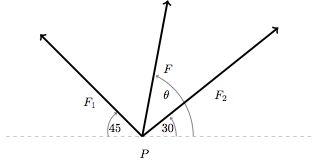# Problem: Two forces F1 and F2 with magnitudes 100 newtons and 120 newtons, respectively, act on an object at point P as shown in the figure (not to scale). Find the magnitude and direction of the resultant.

###### FREE Expert Solution

The magnitude of a 2-dimensional vector:

$\overline{)\mathbf{|}\stackrel{\mathbf{⇀}}{\mathbit{A}}\mathbf{|}{\mathbf{=}}\sqrt{{{\mathbit{A}}_{\mathbit{x}}}^{\mathbf{2}}\mathbf{+}{{\mathbit{A}}_{\mathbit{y}}}^{\mathbf{2}}}}$

Direction of a 2-dimensional vector:

$\overline{){\mathbf{tan}}{\mathbit{\theta }}{\mathbf{=}}\frac{{\mathbit{A}}_{\mathbit{y}}}{{\mathbit{A}}_{\mathbit{x}}}}$

Resolving a 2-dimensional vector into its components:

Let the positive y-axis point upward and the positive x-axis point ot the left.

θ1 = (180 - 45) = 135

94% (255 ratings)###### Problem Details

Two forces F1 and F2 with magnitudes 100 newtons and 120 newtons, respectively, act on an object at point P as shown in the figure (not to scale). Find the magnitude and direction of the resultant.